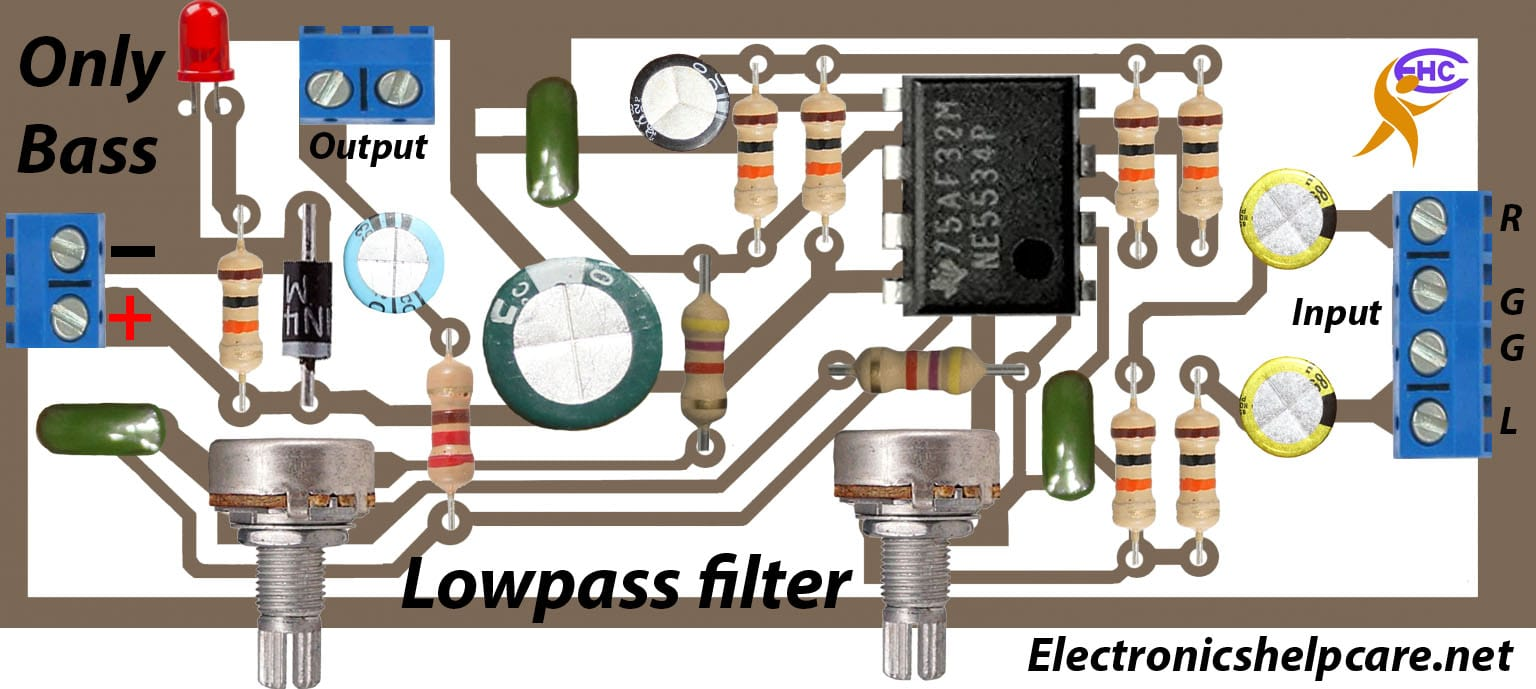# how to make subwoofer circuit?

## Subwoofer Circuit diagram:

This is the Subwoofer circuit diagram for the sub-Speaker. if we want to make only a sub-woofer then we have to use this circuit diagram as a pre amplifier. this circuit diagram makes the audio-only bass. this is the low part for the sound. if we use this circuit diagram in our amplifier then we can not get high or mid sound. we will get only the low part.Here used 12 voltage only. many circuit diagrams used positive Ground and negative voltage.  this circuit we can use only in 12 voltage.

We can see the component list inside the circuit. this circuit is a mono circuit. in this circuit Audio input is stereo and audio output is mono. All resistors are 1/4 watts. and all capacitors are 25 voltages.

If we want to make this circuit diagram

Our another post for you

transistor circuit diagram of 2sa1943 and 2sc5200

Thanks a lot to be with us. If you want another post then please visit our website.

We have another post for you. like as repairing amplifier,